# WhisperFin PRO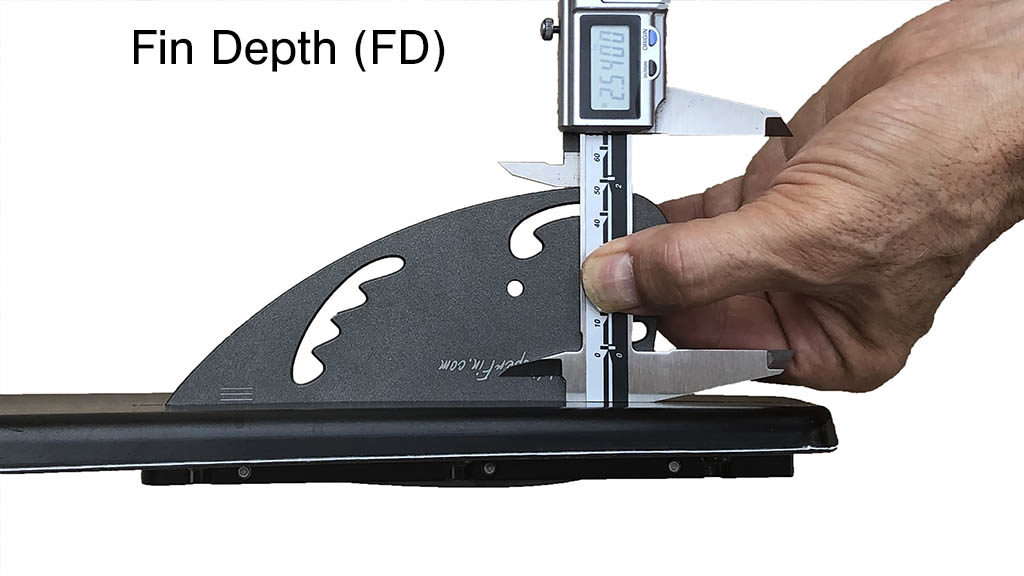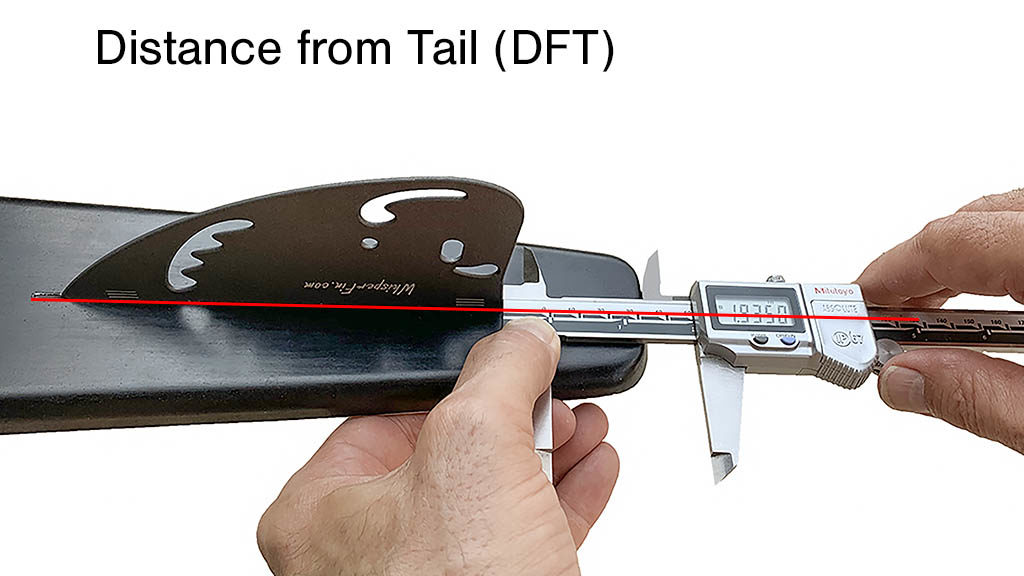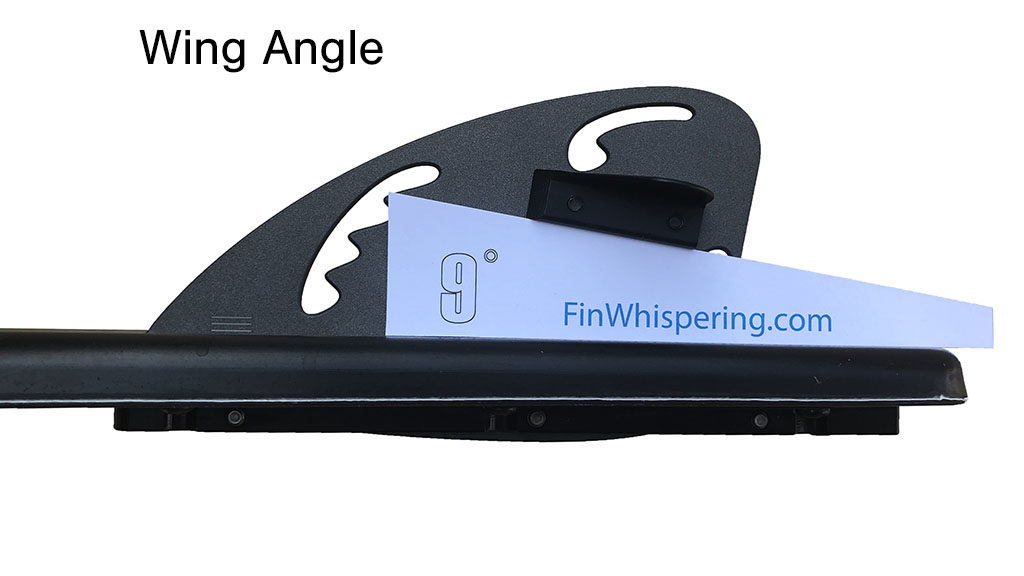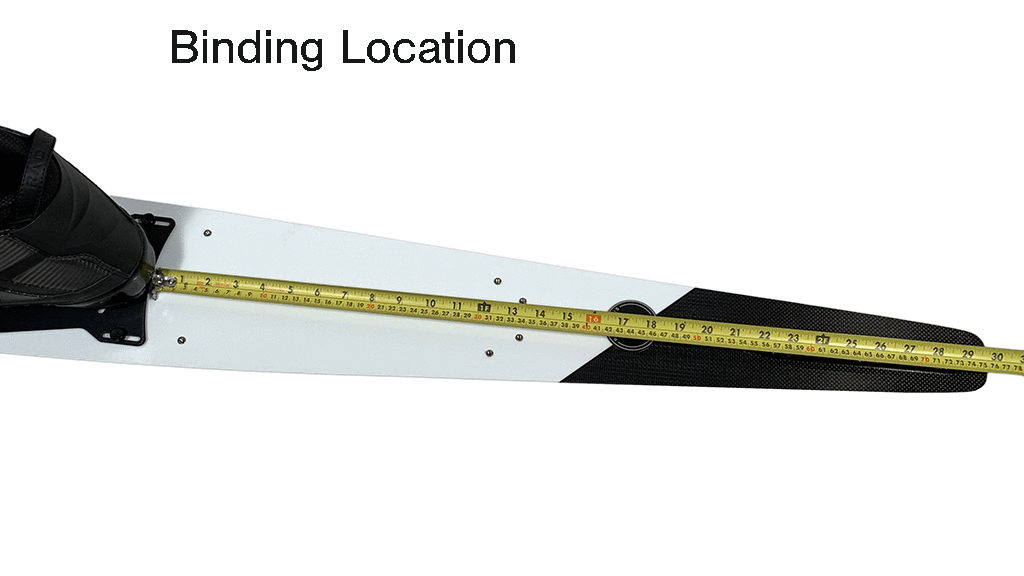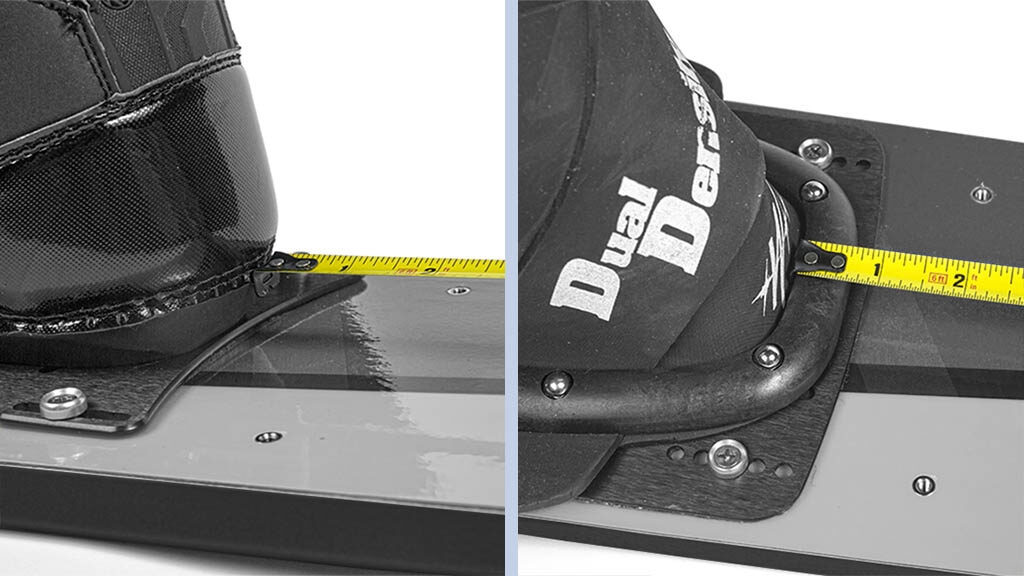The measurements listed in this database were taken using these industry standard techniques

## Conversion Calculator – Original WhisperFin to PRO

If you are using the original WhisperFin and love your setup, this calculator will convert your current settings into WhisperFin PRO settings that will maintain your ski’s balance while introducing PRO fin benefits. Then even if you are happy with your new setup, I encourage you to further explore the potential of your new fin by following the tuning guides that came with your fin.

##### Binding and Wing Settings

Binding and wing settings transfer unchanged from the original WhisperFin to the PRO fin.

V–1.2 (03.24.21): This algorithm may evolve for awhile.

## Starting Setups

These setups are called “starting setups” because they are just that—good starting points from which to develop your own personalized setup. Keep in mind that while these setups worked great for someone, that “someone” may have bindings, technique and stature that are completely different than yours. So whether you start with the generic installation setup that came with your fin, or with one of the following model-specific setups, I still recommend you do a little custom tuning for best personal results. And custom tuning is probably easier than you think. Just follow the tuning instructions that came with your fin, or go to: www.finwhispering.com/whisperfin-tuning

Not every ski built will be listed in these Starting Setups. If your ski isn’t listed, you can start with the setup for another ski of a similar size and character to yours. And if there is a setup listed for your ski but not in your size, adjust the listed setup as follows:

• Move your bindings ½” for every inch of ski length difference (e.g. If the listed ski 1″ larger than yours, move your bindings forward ½”).
• Change DFT .005″ for every inch of ski length difference (e.g. If the listed ski 1″ larger than yours, increase DFT by .005″).

## — work in progress —

Connelly
##### Connelly Installation Note:

With Connelly’s unique new fin block, the adjustment set screws contact the top sheet of the ski before you can achieve the desired FD and FL with the WhisperFin. This is not a problem. You can either adjust the fin by hand without the use of the set screws, Dremel out a round hole through the top sheet for the set screws to pass through (do not use a standard drill bit as it may snag and crack the top sheet). Or you can simply force the set screws through the top sheet into the foam core (this works fine and does no structural or cosmetic damage).

* Notice the significant difference between the 66″ and 67″ DFT measurements. The 67″ setup was for a 35-off skier who was struggling with too much tip-bite. This short DFT strategy will work to reduce tip-grab for any sized ski. And note that it’s not the short DFT that reduces tip-bite, it’s the short leading edge location (LE).

## GT-R

PRO Fin Settings

Nimble
Bf = 28.625
Wing = 8°
FD = 2.510
FL = 5.857
DFT = 1.843
LE = 7.700

Supportive
Bf = 29.125
Wing = 8°
FD = 2.500
FL = 5.847
DFT = 1.798
LE = 7.645

#### 67″

Anti Tip-grab
Bf = 29.625
Wing = 7°
FD = 2.500
FL = 5.847
DFT = 1.698 *
LE = 7.545**
*The front (FL) set screw must be removed to achieve this DFT

**This setup can be made nimbler by increasing DFT by up to .110″  or until the tip starts grabbing

PRO Fin Settings

Supportive
Bf = 29.375
Wing = 7°
FD = 2.480
FL = 5.837
DFT = 1.773
LE = 7.610

PRO Fin Settings

Nimble
Bf = 29.375
Wing = 7°
FD = 2.500
FL = 5.849
DFT = 1.843
LE = 7.692

Supportive
Bf = 29.5
Wing = 7°
FD = 2.510
FL = 5.857
DFT = 1.813
LE = 7.670

Joel Howley
(extra support)
Bf = 29.5
Wing = 9°
FD = 2.525
FL = 5.868
DFT = 1.710
LE = 7.678

D3

Bf = 28.625
Wing = 7°
FD = 2.455
FL = 5.817
DFT = 1.838
LE = 7.655

Bf = 29.25
Wing = 7°
FD = 2.490
FL = 5.842
DFT = 1.813
LE = 7.655

Bf = 29.75
Wing = 7°
FD = 2.500
FL = 5.849
DFT = 1.813
LE = 7.662

Bf = 30.125
Wing = 8°
FD = 2.500
FL = 5.847
DFT = 1.818
LE = 7.665

Bf = 30.625
Wing = 7°
FD = 2.510
FL = 5.857
DFT = 1.813
LE = 7.670

Bf = 29.65
Wing = 7°
FD = 2.495
FL = 5.847
DFT = 1.838
LE = 7.685

Bf = 30.15
Wing = 7°
FD = 2.500
FL = 5.847
DFT = 1.848
LE = 7.695

Bf = 30.65
Wing = 7°
FD = 2.500
FL = 5.847
DFT = 1.843
LE = 7.690

## NRG R2

Bf = 29.65
Wing = 7°
FD = 2.500
FL = 5.850
DFT = 1.838
LE = 7.688

Bf = 30.15
Wing = 7°
FD = 2.505
FL = 5.854
DFT = 1.848
LE = 7.702

#### 68″

Bf = 30.65
Wing = 7°
FD = 2.505
FL = 5.854
DFT = 1.850
LE = 7.704

Goode

Wing Note: The setups here all assume small wing use. But most, if not all, of the current Goodes work best with the large wing run at 1.5° less angle than your normal small wing setting.

Note: These measurements are with the tips (FL) and head (DFT) of a normal caliper, not a slot caliper. FL measurements are very roughly .075″ shorter and DFT measurements about .010″ longer using a slot caliper, but these conversions vary widely depending on ski design.

Bf = 29.5
Wing = 9°
FD = 2.500
FL = 5.850
DFT = 1.723
LE = 7.573

Bf = 29.625
Wing = 9°
FD = 2.500
FL = 5.850
DFT = 1.723
LE = 7.573

Bf = 29.875
Wing = 9.5°
FD = 2.500
FL = 5.845
DFT = 1.733
LE = 7.578

## 9970 CC

Bf = 29.5
Wing = 9° Large
FD = 2.500
FL = 5.849
DFT = 1.703
LE = 7.552

#### 67″

Bf = 29.625
Wing = 9.5° Large
FD = 2.500
FL = 5.849
DFT = 1.703
LE = 7.552

#### 67.75″

Bf = 29.5
Wing = 9.5° Large
FD = 2.495
FL = 5.845
DFT = 1.743
LE = 7.588

#### 68.5″

Bf = 29.875
Wing = 9° Large
FD = 2.495
FL = 5.847
DFT = 1.773
LE = 7.620
This setup is a good estimate

Aggressive
Bf = 28.875
Wing = 9.5°
FD = 2.500
FL = 5.847
DFT = 1.753
LE = 7.600

Less Tip-bite
Bf = 28.875
Wing = 9.5°
FD = 2.500
FL = 5.847
DFT = 1.703
LE = 7.550

Aggressive
Bf = 29.125
Wing = 9°
FD = 2.500
FL = 5.852
DFT = 1.758
LE = 7.610

Less Tip-bite
Bf = 29.125
Wing = 9°
FD = 2.500
FL = 5.852
DFT = 1.713
LE = 7.565

Aggressive
Bf = 29.375
Wing = 9.5°
FD = 2.510
FL = 5.857
DFT = 1.763
LE = 7.620

Less Tip-bite
Bf = 29.375
Wing = 9.5°
FD = 2.510
FL = 5.857
DFT = 1.713
LE = 7.570

## RéVolution

#### 66.5″

Bf = 29.25
Wing = 9°
FD = 2.500
FL = 5.852
DFT = 1.828
LE = 7.680

Excellent tip hold out of turns, but if you experience tip-grab, shorten LE

#### 67.25″

Bf = 29.500
Wing = 9°
FD = 2.500
FL = 5.852
DFT = 1.733
LE = 7.585

Set up to defend against tip-bite from tip-high approach to turns

#### 68″

Bf = 29.625
Wing = 9°
FD = 2.510
FL = 5.852
DFT = 1.773
LE = 7.625

An accomplished Big Dawg’s setup

Aggressive
Bf (N1) = 28.875
Bf (XT) = 28.875
Wing = 9.5°
FD = 2.500
FL = 5.847
DFT = 1.753
LE = 7.600

Less Tip-bite
Bf (N1) = 28.875
Bf (XT) = 28.875
Wing = 9.5°
FD = 2.500
FL = 5.847
DFT = 1.703
LE = 7.550

Aggressive
Bf (N1) = 29
Bf (XT) = 29
Wing = 9°
FD = 2.500
FL = 5.852
DFT = 1.758
LE = 7.610

Less Tip-bite
Bf (N1) = 29
Bf (XT) = 29
Wing = 9°
FD = 2.500
FL = 5.852
DFT = 1.713
LE = 7.565

Aggressive
Bf (N1) = 29.375
Bf (XT) = 29.125
Wing = 9°
FD = 2.510
FL = 5.857
DFT = 1.763
LE = 7.620

Less Tip-bite
Bf (N1) = 29.375
Bf (XT) = 29.125
Wing = 9°
FD = 2.510
FL = 5.857
DFT = 1.713
LE = 7.570

Bf = 28.75
Wing = 9°
FD = 2.505
FL = 5.852
DFT = 1.803
LE = 7.655

Bf = 29
Wing = 9°
FD = 2.510
FL = 5.857
DFT = 1.783
LE = 7.640

Bf = 29.625
Wing = 9° Large
FD = 2.510
FL = 5.857
DFT = 1.778
LE = 7.635

HO

Bf = 28 ⅞
Wing = 8°
FD = 2.450
FL = 5.812
DFT = 1.873
LE = 7.685

Bf = 28.75
Wing = 8°
FD = 2.450
FL = 5.812
DFT = 1.878
LE = 7.690

Bf = 29.25
Wing = 8°
FD = 2.450
FL = 5.812
DFT = 1.883
LE = 7.695

KD

## Platinum

Nimble
Bf = 28.625
Wing = 8°
FD = 2.470
FL = 5.827
DFT = 1.813
LE = 7.640

More Supportive
Bf = 28.625
Wing = 8°
FD = 2.485
FL = 5.837
DFT = 1.808
LE = 7.645

Nimble
Bf = 29.125
Wing = 8°
FD = 2.480
FL = 5.837
DFT = 1.823
LE = 7.660

More Supportive
Bf = 29.25
Wing = 8°
FD = 2.510
FL = 5.857
DFT = 1.793
LE = 7.650

#### 67″

Nimble
Bf = 29.625
Wing = 8°
FD = 2.465
FL = 5.824
DFT = 1.786*
LE = 7.610
* Lengthen DFT until tip grabs

More Supportive

Bf = 29.75
Wing = 8°
FD = 2.500
FL = 5.847
DFT = 1.808
LE = 7.655

Lapoint

For more customized tuning guidance see: WhisperFin Ski-tuning –101 and the Whisperfin Ski-tuning Flowchart

## LP1

Bf = 29.25
Wing = 7°
FD = 2.505
FL = 5.853
DFT = 1.840
LE = 7.693

Bf = 29.75
Wing = 8°
FD = 2.510
FL = 5.858
DFT = 1.842
LE = 7.700

#### 68″

Bf = 30.25
Wing = 8°
FD = 2.510
FL = 5.858
DFT = 1.852
LE = 7.710

Nimble – Start with these settings if you are light, or consider yourself a finesse skier. The more nimble your setup can be, the better the tip hold will be exiting turns and during the cut. Your setup is too nimble if the tail feels slippery at the end of turns.

More Supportive – Start with these settings if you consider yourself a powerful skier who tends to force the ski, if you tend to get tip-grab, or if the nimble settings make the tail feel too slippery.

These two setups are NOT at extreme ends of the setup spectrum. If anything, they bracket the middle third. So experimenting with either of these setups is encouraged. If you want more or less tail grip make the fin deeper or shallower. And it’s always a good idea to customize yawing tip-engagement by moving the fin back and forth (forward for more tip engagement and back for less). You want to be as far forward as your technique will allow before the tip starts grabbing occasionally.

For more customized tuning guidance see: WhisperFin Ski-tuning –101 and the Whisperfin Ski-tuning Flowchart

Bf = 28.625
Wing = 8°
FD = 2.410
FL = 5.782
DFT = 1.863
LE = 7.645

Bf = 31.125
Wing = 8°
FD = 2.490
FL = 5.842
DFT = 1.843
LE = 7.685

Bf = 29.25
Wing = 9°
FD = 2.480
FL = 5.827
DFT = 1.843
LE = 7.670

Nimble
Bf = 29.625
Wing = 9°
FD = 2.480
FL = 5.832
DFT = 1.853
LE = 7.685

More Stable
Bf = 29.75
Wing = 9°
FD = 2.510
FL = 5.857
DFT = 1.813
LE = 7.670

Nimble
Bf = 30.125
Wing = 9°
FD = 2.480
FL = 5.832
DFT = 1.850
LE = 7.682

More Stable
Bf = 30.25
Wing = 9°
FD = 2.500
FL = 5.847
DFT = 1.828
LE = 7.675

Nimble
Bf = 30.75
Wing = 9°
FD = 2.480
FL = 5.837
DFT = 1.863
LE = 7.700

More Stable
Bf = 30.875
Wing = 9°
FD = 2.500
FL = 5.852
DFT = 1.833
LE = 7.685

Bf = 29.625
Wing = 8°
FD = 2.480
FL = 5.817
DFT = 1.888
LE = 7.705

Bf = 30.75
Wing = 8°
FD = 2.480
FL = 5.837
DFT = 1.853
LE = 7.690

Nimble
Bf = 32.0
Wing = 8°
FD = 2.480
FL = 5.835
DFT = 1.865
LE = 7.700

More Stable
Bf = 32.0
Wing = 8°
FD = 2.495
FL = 5.847
DFT = 1.838
LE = 7.685

Bf = 32.5
Wing = 8°
FD = 2.490
FL = 5.842
DFT = 1.838
LE = 7.680